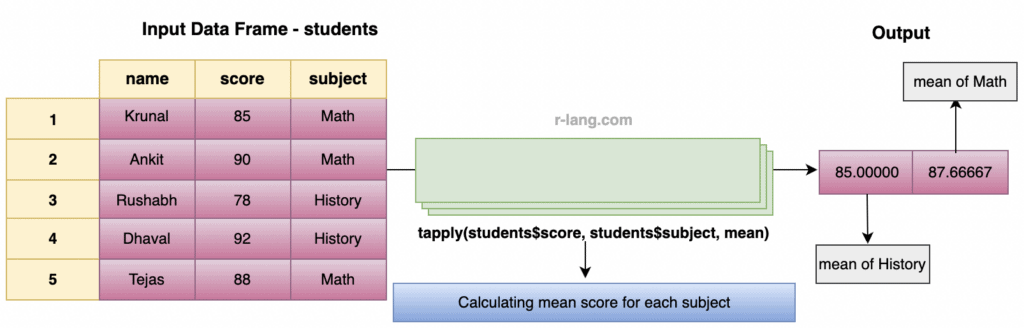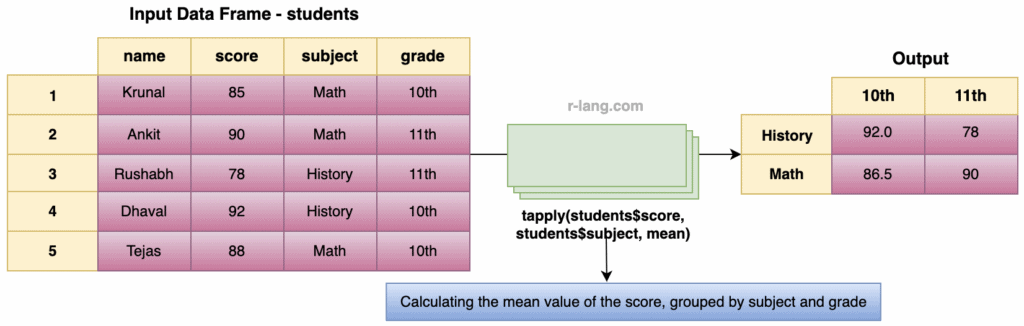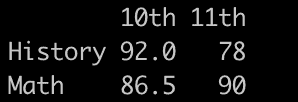# R tapply() Function

The tapply() function is used to apply a function to subsets of a vector, categorized by some factors.

## Syntax

``tapply(INPUT, INDEX, FUN = NULL, ..., simplify = TRUE)``

## Parameters

1. INPUT: It is a numeric or character vector.
2. INDEX: It is a factor or a list of factors.
3. FUN: It is the function to be applied.
4. …: They are arguments to FUN.
5. simplify: It is a logical argument. If TRUE, the result is simplified to the lowest possible dimension.

## Example 1: How to use the tapply() function

Let’s apply the tapply() function to the data frame of student scores in different subjects and calculate the mean score for each subject.``````students <- data.frame(
name = c("Krunal", "Ankit", "Rushabh", "Dhaval", "Tejas"),
score = c(85, 90, 78, 92, 88),
subject = c("Math", "Math", "History", "History", "Math")
)

# Calculate mean score for each subject
tapply(students\$score, students\$subject, mean)``````

Output

`````` History    Math
85.00000   87.66667
``````

## Example 2: Using multiple factors

Let’s calculate the mean value of the score, grouped by subject and grade:You can see from the figure that we are calculating the mean value of score grouped by subject and grade using the tapply() function.

``````students <- data.frame(
name = c("Krunal", "Ankit", "Rushabh", "Dhaval", "Tejas"),
score = c(85, 90, 78, 92, 88),
subject = c("Math", "Math", "History", "History", "Math"),
grade = c("10th", "11th", "11th", "10th", "10th")
)

# Calculate mean score for each subject and grade combination
``````

Output## Example 3: Using additional arguments

You can also pass additional arguments to the function you are applying.

Let’s say you want to calculate trimmed means for each subject:

``````students <- data.frame(
name = c("Krunal", "Ankit", "Rushabh", "Dhaval", "Tejas"),
score = c(85, 90, 78, 92, 88),
subject = c("Math", "Math", "History", "History", "Math"),
grade = c("10th", "11th", "11th", "10th", "10th")
)

# Calculate trimmed mean (trimming 10%) for each subject
tapply(students\$score, students\$subject, mean, trim = 0.1)``````

Output

`````` History      Math
85.00000     87.66667``````

You need to remember that the result of the tapply() function will be a table or array, depending on the number of factors.

If you only have one factor, the result will be a named vector.

If you have multiple factors, the result will be a multi-dimensional array.

The tapply() function is helpful for quick and simple aggregations without needing more complex data manipulation packages.

However, for more complex data manipulation tasks, packages like dplyr or data.table might be more suitable.